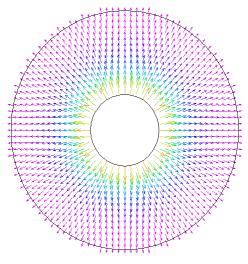﻿ Sample Problems > Applications > Stress > prestube

# prestube

Navigation:  Sample Problems > Applications > Stress >

# prestube{ PRESTUBE.PDE

This example models the stress in a tube with an internal pressure.

-  from "Fields of Physics on the PC"  by Gunnar Backstrom

}

 title   ' Tube With Internal Pressure' variables   u   v definitions   mm = 1e-3   r1 = 3*mm             r2 = 10*mm             q21= r2/r1   mu = 0.3   E = 200e9       {Steel}   c = E/(1-mu^2)         G = E/2/(1+mu)   dabs= sqrt(u^2+ v^2)   ex= dx(u)             ey= dy(v)                 exy= dx(v)+ dy(u)   sx= c*(ex+ mu*ey)     sy= c*(mu*ex+ ey)         sxy= G*exy   p1= 1e8         { the internal pressure }   { Exact expressions }   rad= sqrt(x^2+ y^2)   sr_ex= -p1*((r2/rad)^2 - 1)/(q21^2 - 1)   st_ex=  p1*((r2/rad)^2 + 1)/(q21^2 - 1)   dabs_ex= abs( rad/E*(st_ex- mu*sr_ex))equations               { Constant temperature, no volume forces }

u:   dx( c*(dx(u) + mu*dy(v)) ) + dy( G*(dx(v)+ dy(u)) )= 0

v:   dx( G*( dx(v)+ dy(u)) )+  dy( c*(dy(v) + mu*dx(u)) )= 0

constraints             { Since all boundaries are free, it is necessary

to apply constraints to eliminate rigid-body motions }

integral(u) = 0

integral(v) = 0

integral(dx(v)-dy(u)) = 0

boundaries

region 1

start (r2,0)

load(u)= 0           { Outer boundary is free }

arc to (0,r2) to (-r2,0) to (0,-r2) to close

start (r1,0)                 { Cut-out }

load(u)= p1*x/r1     { Normal component of x-stress }

load(v)= p1*y/r1     { Normal component of y-stress }

arc to (0,-r1) to (-r1,0) to (0,r1) to close

monitors

contour(dabs)

plots

grid(x+200*u, y+200*v)

elevation(sx, sr_ex) from (r1,0) to (r2,0)

elevation(sy, st_ex) from (r1,0) to (r2,0)

contour(dabs)       contour((dabs-dabs_ex)/dabs_ex)

contour(u)           contour(v)

vector(u,v)         vector(u/dabs, v/dabs)

contour(sx)         contour(sy)             contour(sxy)

end